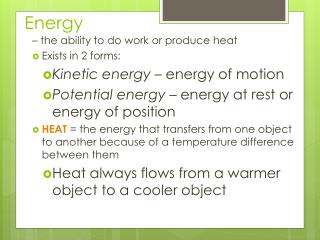DownloadDownload PresentationEnergy

# Energy

Download Presentation## Energy

- - - - - - - - - - - - - - - - - - - - - - - - - - - E N D - - - - - - - - - - - - - - - - - - - - - - - - - - -
##### Presentation Transcript

1. Energy – the ability to do work or produce heat • Exists in 2 forms: • Kinetic energy – energy of motion • Potential energy – energy at rest or energy of position • HEAT= the energy that transfers from one object to another because of a temperature difference between them • Heat always flows from a warmer object to a cooler object

2. Energy • Kinetic energy – in a chemical reaction temperature is the determining factor • The higher the temperature…the faster the particles move…the higher the average kinetic energy • Therefore, the lower the temperature the ________ the kinetic energy. • Temperature is a measure of the average kinetic energy • Kelvin scale: 0 K = -273 °C, °C + 273 = K

3. Law of Conservation of Energy • Law of Conservation of Energy – Energy is neither created nor destroyed

4. Heat (q) • Heat or energy can be in joules, calories, kilocalories, or kilojoules • The SI unit is the joule • 1 Cal = 1000 cal = 1 kcal • 1 cal = 4.186 J • 1kcal = 4186J • 1 J = 0.239 cal

5. Heat Capacity • The amount of heat it takes to change an object’s temperature by 1ºC • Depends on an object’s mass • Ex. A cup of water has a greater heat capacity than a drop of water.

6. Specific Heat (C) • Specific Heat (C) – the amount of heat required to raise the temperature of 1 gram of a substance by 1C • Specific heat is an intensive property, and therefore does not depend on size • Every substance has its own specific heat (look at your CRM) • Ex. Water = 4.184 J/(gºC) Glass = 0.500 J/(gºC) *The higher the specific heat, the greatest amount of energy! The lower the specific heat, the larger the increase in temperature!

7. Specific Heat • Units for C = J/gºC (joules per gram degree Celsius) • Equation for Specific Heat: C = q / (m Δ T) • C = specific heat; • q = heat; • m = mass • ΔT = change in temperature • This equation can be rearranged to solve for heat (q) q= mCΔT

8. Specific Heat • A 10.0 g sample of iron changes temperature from 25.0C to 50.4 C while releasing 114 joules of heat. Calculate the specific heat of iron.

9. Example • C= q/ (m∆T) • C=114 J/ (10.0 g x 25.4°C) • C = 0.45 J/g C

10. Yet another example • 4.50 g of a gold nugget absorbs 276 J of heat. What is the final temperature of the gold if the initial temperature was 25.0 C & the specific heat of the gold is 0.129J/g C

11. Yet another example • C= q/ (m∆T); rearrange to find • ∆T = q / (C x m) • ∆T = 276 J / (.129 J/g°C x 4.50 g) • T = 475.45C • T = Tf-Ti • 475.45 = Tf-25 • Tf = 500.45 C